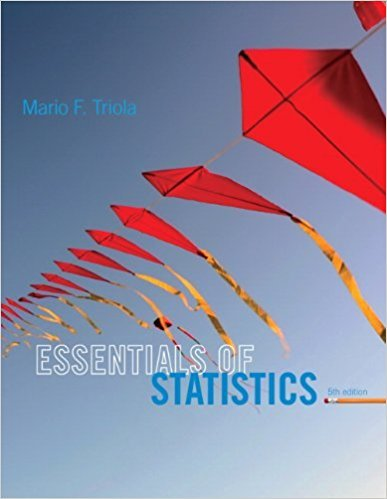×
×

# Solutions for Chapter 10.3: Regression## Full solutions for Essentials of Statistics | 5th Edition

ISBN: 9780321924599Solutions for Chapter 10.3: Regression

Solutions for Chapter 10.3
4 5 0 371 Reviews
19
5
##### ISBN: 9780321924599

This expansive textbook survival guide covers the following chapters and their solutions. Since 34 problems in chapter 10.3: Regression have been answered, more than 14424 students have viewed full step-by-step solutions from this chapter. Essentials of Statistics was written by and is associated to the ISBN: 9780321924599. This textbook survival guide was created for the textbook: Essentials of Statistics, edition: 5. Chapter 10.3: Regression includes 34 full step-by-step solutions.

Key Statistics Terms and definitions covered in this textbook

A formula used to determine the probability of the union of two (or more) events from the probabilities of the events and their intersection(s).

• All possible (subsets) regressions

A method of variable selection in regression that examines all possible subsets of the candidate regressor variables. Eficient computer algorithms have been developed for implementing all possible regressions

• Asymptotic relative eficiency (ARE)

Used to compare hypothesis tests. The ARE of one test relative to another is the limiting ratio of the sample sizes necessary to obtain identical error probabilities for the two procedures.

• Backward elimination

A method of variable selection in regression that begins with all of the candidate regressor variables in the model and eliminates the insigniicant regressors one at a time until only signiicant regressors remain

• Bayes’ estimator

An estimator for a parameter obtained from a Bayesian method that uses a prior distribution for the parameter along with the conditional distribution of the data given the parameter to obtain the posterior distribution of the parameter. The estimator is obtained from the posterior distribution.

• Box plot (or box and whisker plot)

A graphical display of data in which the box contains the middle 50% of the data (the interquartile range) with the median dividing it, and the whiskers extend to the smallest and largest values (or some deined lower and upper limits).

• C chart

An attribute control chart that plots the total number of defects per unit in a subgroup. Similar to a defects-per-unit or U chart.

• Conditional probability distribution

The distribution of a random variable given that the random experiment produces an outcome in an event. The given event might specify values for one or more other random variables

• Continuous distribution

A probability distribution for a continuous random variable.

• Contour plot

A two-dimensional graphic used for a bivariate probability density function that displays curves for which the probability density function is constant.

• Control limits

See Control chart.

• Covariance

A measure of association between two random variables obtained as the expected value of the product of the two random variables around their means; that is, Cov(X Y, ) [( )( )] =? ? E X Y ? ? X Y .

• Defect

Used in statistical quality control, a defect is a particular type of nonconformance to speciications or requirements. Sometimes defects are classiied into types, such as appearance defects and functional defects.

• Defect concentration diagram

A quality tool that graphically shows the location of defects on a part or in a process.

• Design matrix

A matrix that provides the tests that are to be conducted in an experiment.

• Distribution free method(s)

Any method of inference (hypothesis testing or conidence interval construction) that does not depend on the form of the underlying distribution of the observations. Sometimes called nonparametric method(s).

• Erlang random variable

A continuous random variable that is the sum of a ixed number of independent, exponential random variables.

• Error propagation

An analysis of how the variance of the random variable that represents that output of a system depends on the variances of the inputs. A formula exists when the output is a linear function of the inputs and the formula is simpliied if the inputs are assumed to be independent.

• Exhaustive

A property of a collection of events that indicates that their union equals the sample space.

• False alarm

A signal from a control chart when no assignable causes are present

×